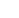﻿ Blind Source Separation - Research - Yoav Schechner

# Yoav Y. Schechner: Research### Blind Source SeparationDifferential entropy is a quantity used in many signal processing problems. Often we need to calculate not only the entropy itself, but also its gradient with respect to various variables, for efficient optimization, sensitivity analysis, etc. Entropy estimation can be based on an estimate of the probability density function, which is computationally costly if done naively. Some prior algorithms use computationally efficient non-parametric entropy estimators. However, differentiation of the previously proposed estimators is difficult and may even be undefined.

To counter these obstacles, we consider non-parametric kernel entropy estimation that is differentiable. We present two different accelerated kernel algorithms. The first accelerates the entropy gradient calculation based on a back propagation principle. It allows calculating the differential entropy gradient in the same complexity as that of calculating the entropy itself. The second algorithm accelerates the estimation of both entropy and its gradient by using fast convolution over a uniform grid. We expand this approach to fast optimization of mutual information (MI), and then apply it to BSS by independent component analysis (ICA). This yields both high separation performance and low computational complexity. For K sources with N samples, our ICA algorithm has an iteration complexity of at most O(KNlogN + K2N).

In our work about "convolutive mixtures" we are inspired by mixtures occurring in photgraphy, microscopy and tomography. Their formation process involves focusing on an object layer, over which defocused layers are superimposed. BSS of convolutive image mixtures by direct optimization of MI is very complex and suffers from local minima. Thus, we devise an efficient approach to solve these problems. The convolutive BSS problem is converted into a set of instantaneous (pointwise) problems, using a short time Fourier transform (STFT). Standard BSS solutions for instantaneous problems suffer, however, from scale and permutation ambiguities. We obtain diambiguition by exploiting a parametric model of the defocus point spread function. Moreover, we enhance the efficiency of the approach by exploiting the sparsity of the STFT representation as a prior.

We have also applied similar principles in order to overcome haze. I this case, a major problem is spatially-varying reduction of contrast by stray radiance (airlight), which is scattered by the haze particles towards the camera. We blindly estimate an airlight parameter, thus enabing blind airlight separation based on polarization-filtered images. We avoid common ICA ambiguities by a physics-based model.

## Publications

1. Sarit Shwartz, Michael Zibulevsky and Yoav Y. Schechner, “Fast kernel entropy estimation and optimization,” Signal Processing, Vol. 85, No. 5, pp. 1045-1058 (2005).
2. Sarit Shwartz, Michael Zibulevsky and Yoav Y. Schechner, “ICA using kernel entropy estimation with NlogN complexity,” Proc. ICA - Int. Conf. on Independent Component Analysis and Blind Signal Separation, pp. 422-429 (2004).
3. Sarit Shwartz, Yoav Y. Schechner and Michael Zibulevsky, “Efficient separation of convolutive image mixtures,” Proc. ICA - Int. Conference on Independent Component Analysis and Blind Signal Separation, pp. 246-253 (2006).
4. Sarit Shwartz, Einav Namer and Yoav Y. Schechner, “Blind haze separation,” Proc. IEEE CVPR, Vol. 2, pp. 1984-1991 (2006).
5. Sarit Shwartz, Yoav Y. Schechner and Michael Zibulevsky, “Blind separation of convolutive image mixtures,” Neurocomputing, Vol. 71, pp. 2164-2179 (2008), Special issue on Advances in Blind Signal Processing.

## Presentations

1. NlogN Entropy Optimization” (1.8 Mb, PowerPoint)

## Software

Download the code for efficient non-parametric ICA optimization. The archive contains the following files: